# Pandas DataFrame Plot pie graph

Pandas plot

Pandas.DataFrame.plot to generate pie using data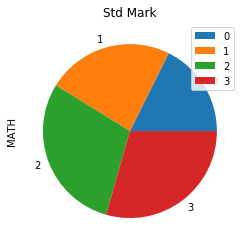Python Pandas Plot Pie chart by using DataFrame with options & save as image
``````import pandas as pd
my_dict={
'NAME':['Ravi','Raju','Alex','Ronn'],
'MATH':[30,40,50,50]
}
df = pd.DataFrame(data=my_dict)
df.plot.pie(title="Std Mark",y='MATH')``````

## pie chart with options

There are several options we can add to above pie diagram.

## figsize :Size of the graph , it is a tuple saying width and height in inches, figsize=(6,3). Here width is 6 inches and height is 3 inches. Since our pia chart is a circle so better to use equal width and height.
``df.plot.pie(title="Std Mark",y='MATH',figsize=(4,4))``

## fontsize

fontsize=20 , we can set the font size used labels in x and y axis.``````df.plot.pie(title="Std Mark",
y='MATH',fontsize=20)``````

## labels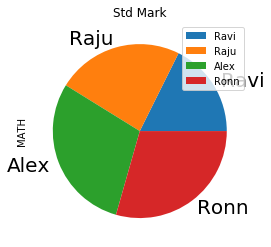We can define our labels by using list.
``````my_labels=['Ravi','Raju','Alex','Ronn']
df.plot.pie(title="Std Mark",y='MATH',
fontsize=20,labels=my_labels)``````

## labeldistance

Distance of Label from center of the Pie chart, default is 1.1 (float ).

## legend

Default value is True, we can remove by using `legend=False`

## explode

We can add option to explode one segment by using a tuple.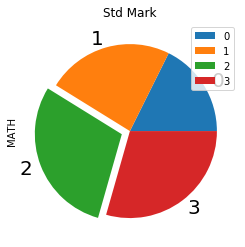``````my_explode=(0,0,0.1,0)
df.plot.pie(title="Std Mark",y='MATH',
fontsize=20,explode=my_explode)``````

We can change the value ( from 0 ) to move the segments more distance. Here we are moving the first segment more.``````my_explode=(1.5,0.0,0.1,0)
df.plot.pie(title="Std Mark",
y='MATH',explode=my_explode)``````

## startangle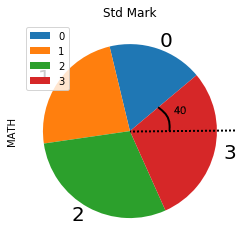We can add option startangle=40 to give the initial starting point for the first segment. 0 degree is the base from which anti clockwise the angle is checked.
``````df.plot.pie(title="Std Mark",y='MATH',
fontsize=20,startangle=40)``````

## counterclock

Default value is True. The direction of fractions.

## rotatelabels

Default is False, Rotate each label matching to slice in degree.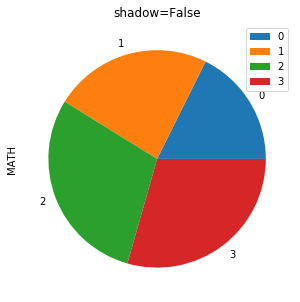``````df.plot.pie(title="shadow=False",y='MATH',

## colors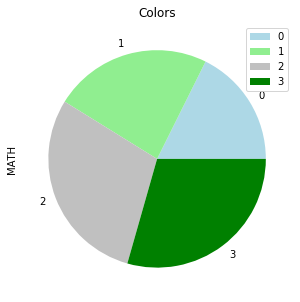We can use the option colors to give different colors to segments. We can use one tuple to define the colours.
``````my_colors=['lightblue','lightgreen',
'silver','green']
df.plot.pie(title="Colors",y='MATH',
figsize=(5,5),colors=my_colors)``````

## autopct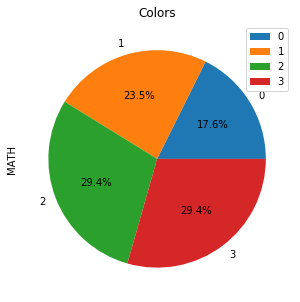To display the percentage of each segment we can define the format. Here is one sample to show one decimal place autopct='%1.1f%%' , for two decimal places it can be autopct='%1.2f%%'
``````df.plot.pie(title="Colors",y='MATH',
autopct='%1.1f%%')``````

## frame

Axes frame is added with the chart if True. Default value is False

## Saving graph as image

``````plot=df.plot.pie(title="Colors",y='MATH',autopct='%1.1f%%')
fig = plot.get_figure()
fig.savefig("D:\\my_data\\output2.png")``````

Subscribe to our YouTube Channel here

## Subscribe

* indicates required
Subscribe to plus2netplus2net.com